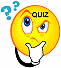Professor Freedman's
Prealgebra ONLINE Homework Schedule
Fall 2023
(15 week course) 8/30/23 - 12/18/23

ONLINE Text: Prealgebra by Miller, O'Neill & Hyde, 3rd Edition, McGrawHill, Inc.,2020

Dates Subject to Change

Assignments

WEEK of 8/30//23

Homework Assigned

Watch the following videos:

Introduction to Algebra -Exponents, Absolute Values & Order of Operations

Addition & Subtraction of Signed Numbers

Complete in Aleks.com through CANVAS Assignments #1 & 2 & 2A

Now complete quiz #1 in Aleks.com through CANVASQUIZ #1 in Aleks.com through CANVAS on the first 2 videos

Homework Tips (Week 1)

WEEK of 9/4

Homework Assigned

Watch the following videos:

Multiplication & Division of Signed Numbers

Order of Operations on Signed Numbers

Evaluating an Algebraic Expression

Commutative, Associative & Distributive Properties

Now complete Assignment #3 and quiz #2 in Aleks.com through CANVASQUIZ #2 in Aleks.com through CANVAS on the Multiplication & Division of Signed Numbers, Evaluating an Algebraic Expression and Distributive Property

Homework Tips (Week 2)

WEEK of 9/11

 Combining Like Terms & Solving Equations(Part 1) Homework Assigned: Watch the following videos: Solving Linear Equations (Part 1) Complete Assignment #4 (Combining Like Terms) and #5 (Equations Part 1) and #6 (Equations Part 2) , #6 (Equations Party 2)QUIZ #3 in Aleks.com through CANVAS

WEEK of 9/18

Homework Assigned:
Watch the following videos:

Proper & Improper Fractions & Mixed Numbers

Converting Mixed Numbers to Improper Fractions

Simplifying Fractions

Multiplying Fractions

Dividing Fractions

Complete Assignments #7, #8 , #9 , #10 (Practice Test) in Aleks.com through CANVASQUIZ #4 and PRACTICE TEST (Homework #10) in Aleks.com through CANVAS

WEEK of 9/25

MAJOR TEST in Aleks.com through CANVAS on Chapters,1,2,3 and part of 4

WEEK of 10/2

Homework Assigned:

Watch the following videos:

Writing Equivalent Fractions

Ordering Fractions

Adding & Subtracting Fractions with Same Denominator

Adding & Subtracting Fractions with Different Denominators,

Multiplication of Mixed Numbers,

Division of Mixed Numbers,

In Aleks through Canvas Complete
Assignment #11 (Equivalent Fraction and Ordering Fractions)

Assignment #12 (Add and Subtract Fractions)
Assignment #13 ( Multiplication & Division of Mixed Numbers

WEEK of 10/9

Homework Assigned:

Watch the following videos:

Subtraction of Mixed Numbers

In Aleks through Canvas Complete:
Assignment #15 (Subtraction of Mixed Numbers with Borrowing)
Assignment #16 (Order of Operations with fractions)QUIZ #5 on MIxed Numbers and Order of Operations with Fractions

WEEK of 10/16

Homework Assigned:
Watch the following videos:

Rounding Decimals,

Assignment #17 (Decimal Notation, Rounding, Ordering, Adding Decimals)
Assignment #18 (Practice Midterm Exam)

Assignment #18 (Practice Test) STUDY FOR MIDTERM EXAM - DO PRACTICE TEST

WEEK of 10/23

MIDTERM EXAM in Aleks.com through CANVAS on Chapters,2,3,4 and Rounding and Ordering Decimals

WEEK of 10/30

Homework Assigned:

Watch the following videos:

Decimals - Multiplication & Division by Powers of Ten

Changing Fractions to Decimals & Decimals to Fractions

In Aleks.com through CANVAS Complete Assignment #19 (Multiplying and Dividing by Powers of 10 and Rounding Quotients) , Assignment #20 (Writing Fractions to Decimals and Decimals to Fractions), Assignment 21 (Mean, Median, Mode), Assignment 22 (Rate & Unit Rate)

WEEK of 11/6

Homework Assigned:

Watch the following videos:

Solving Proportion Word Problems

Assignment #23 (Solving Proportions),Complete #24 (Solving Proportion Word Problems)QUIZ #6 in Aleks.com through CANVAS On Proportions

WEEK of 11/13

Watch the following videos:

Converting Percents to Decimals & Decimals to Percents

Solving Percent Problems Using the Proportion Method

Complete Assignment #25 (Percents, Fractions & Decimals)
Assignment #26 (Solving Little Percent Word Problems by the Proportion Method)
,Assignment #27 ( Solving Bigger Percent Problems),

AND Assignment #28( Practice Test on Proportions & Percents - Study Guide)

WEEK of 11/20

Homework Assigned:
Watch the following video: Plotting Points

Aleks.com through CANVAS
Complete Assignment #29 (Plotting Points)

MAJOR TEST on PROPORTIONS & PERCENTS

WEEK of 11/27

Complete Assignment #30 PRACTICE FINAL EXAM (STUDY GUIDE)

WEEK of 12/4

Aleks.com through CANVAS: Complete Final Exam